### Polynomials Class 10th Mathematics Gujarat Board Solution

##### Question 1.State whether the following statements are true or false:(1)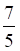is a zero of the linear polynomial p(x) = 5x + 7.(2) p(x) = x2 + 2x + 1 has two distinct zeros.(3) The cubic polynomial p(x) = x3 + x2 — x — 1 has two distinct zeros.(4) The graph of the cubic polynomial p(x) = x3 meets the X—axis at only one point.(5) Any quadratic polynomial p(x) has at least one zero, x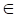RAnswer:(1) p(x) = 5x + 7∴ p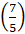= 5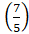+ 7 = 14⇒ p≠0⇒is not a zero of p(x).So the statement is False.(2) Given, p(x) = x2 + 2x + 1To find the zeros of p(x), let p(x) = 0∴ x2 + 2x + 1 = 0∴ (x + 1)2 = 0 Using the identity: (a+ b) 2 = (a2 + 2ab + b2)∴ x + 1 = 0 or x = –1∴ x = –1 or x = –1Here, both the zeros are equal, i.e. –1, and hence not distinct.So the statement is False.(3) Given, p(x) = x3 + x2 — x — 1To find the zeros of p(x), let p(x) = 0∴ x3 + x2 — x — 1 = 0∴ x2 (x + 1) – 1 (x + 1) = 0∴ (x2 – 1)(x + 1) = 0∴ (x – 1) (x + 1)(x + 1) = 0 Using the identity: (a2 – b2) = (a – b) (a + b)∴ x – 1 = 0 or x + 1 = 0 or x + 1 = 0∴ x = 1 or x = –1 or x = –1∴ Two distinct zeros of p(x) are 1 and –1.Hence, p(x) has at the most two distinct zeros.So the statement is True.(4) Given, p(x) = x3To find the zeros of p(x), let p(x) = 0∴x3 = 0⇒ x = 0.⇒ The graph of p(x) = x3 meets the X-axis at only one point i.e. (0, 0).So the statement is True.(5) If the graph of the quadratic polynomial p(x) does not intersect the x-axis at any point, then the quadratic polynomial does not have any real zero.So the statement is False.Question 2.Find the zeros and number of zeros of p(x) = x2 + 9x + 18. Show them on a graph.Answer:Here, p(x) = x2 + 9x + 18To find the zeros of p(x), let p(x) = 0.∴ x2 + 9x + 18 = 0∴ x2 + 6x + 3x + 18 = 0∴ x(x + 6) + 3(x + 6) = 0∴ (x + 6)(x + 3) = 0∴ x = –6 or x = –3Thus, the number of zeros of p(x) = 2.To draw the graph of this polynomial, consider the following values of x and corresponding values of p(x):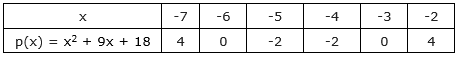On plotting the above points on a graph paper we obtain the following shape of the graph: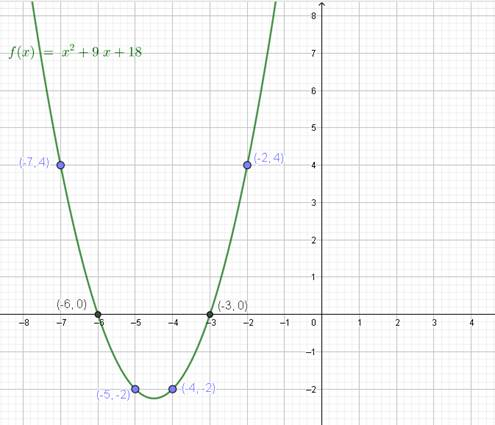Question 3.Find the zeros, the sum and the product of zeros of p(x) = 4x2 + 12x + 5.Answer:Given, p(x) = 4x2 + 12x + 5= 4x2 + 10x + 2x + 5= 2x(2x + 5) + 1(2x + 5)= (2x + 5)(2x + 1)To find the zeros of p(x), put p(x) = 0∴ (2x + 5)(2x + 1) = 0∴ x = –or x = –The zeros of p(x) are –and –.Now, sum of zeros =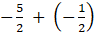=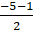= –3and, product of zeros = –× –=Question 4.—4 and 9 are the sum and product of the zeros respectively of a quadratic polynomial. Find the quadratic polynomial.Answer:Let α and β be the zeros of the polynomial p(x) = ax2 + bx + c.Given, α + β = –4 ; αβ = 9A quadratic polynomial with respect to its roots is given by:p(x) = x2 - (α + β) x + αβ = 0Putting the values in this equation we get,p(x) = x2 - (- 4) x + 9p(x) = x2 + 4x + 9Question 5.Find q(x) and r(x), for the quadratic polynomial p(x) = 11x — 21 + 2x2 when divided by 1 + 2xAnswer:Here, dividend polynomial = p(x) = 11x — 21 + 2x2 = 2x2 + 11x — 21and divisor polynomial = s(x) = 1 + 2x = 2x + 1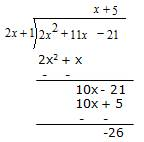The quotient polynomial q(x) = x + 5 and the reminder polynomial r(x) = –26.Question 6.Divide 2x3 + 3x2 — 11x — 6 by x2 + x — 6Answer:Here, dividend polynomial = p(x) = 2x3 + 3x2 — 11x — 6and divisor polynomial = s(x) = x2 + x — 6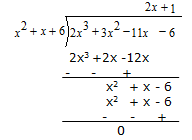Thus, the quotient polynomial q(x) = 2x + 1 and the reminder polynomial r(x) = 0.Question 7.4 is a zero of the cubic polynomial p(x) = x3 — 3x2 — 6x + 8. Find the remaining zeros of p(x).Answer:Given, 4 is a zero of polynomial p(x).So (x – 4) is the factor of p(x).Here, dividend polynomial = p(x) = x3 — 3x2 — 6x + 8and divisor polynomial = s(x) = x – 4.Coefficients of x3, x2, x and x° are 1, –3, –6 and 8 respectively.Taking x – 4 = 0 we get x = 4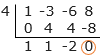∴ p(x) = x3 — 3x2 — 6x + 8= (x – 4) (x2 + x – 2)= (x – 4) (x2 – x + 2x – 2)= (x – 4) (x(x – 1) + 2(x –1))= (x – 4)(x + 2)(x – 1)To find the remaining zeros, let p(x) = 0i.e. (x – 4)(x + 2)(x – 1) = 0∴ The remaining zeros of p(x) are –2 and 1.Question 8.The product of two polynomials is 3x4 + 5x3 — 21x2 — 53x — 30. If one of them is x2 — x — 6, find the other polynomial.Answer:Here p(x) = the dividend polynomial = 3x4 + 5x3 — 21x2 — 53x — 30s(x) = the divisor polynomial = x2 — x — 6Now,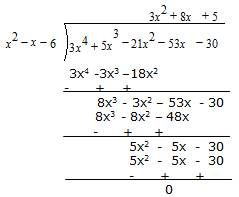∴ The quotient polynomial q(x) = 3x2 + 8x + 5 and the remainder polynomial r(x) = 0.∴ The other polynomial is 3x2 + 8x + 5.Question 9.2 + √3 and 2 — √3 are the zeros of p(x) = x4 — 6x3 — 26x2 + 138x — 35. Find the remaining zeros of p(x).Answer:Given, 2 + √3 and 2–√3 are zeros of p(x).⇒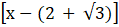and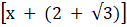are the factors of p(x).Thus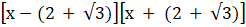= (x2 – 4x + 4 –3) = (x2 – 4x + 1) is also a factor of p(x).To find the remaining zeros, we find the remaining factors using the division process.Here, dividend polynomial = p(x) = 2x4 + 7x3 — 8x2 — 14x + 8and divisor polynomial = s(x) = x2 – 2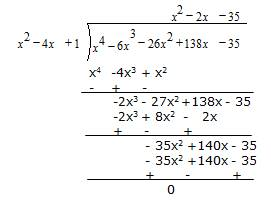⇒ p(x) = 2x4 + 7x3 — 8x2 — 14x + 8 = (x2 – 4x + 1)(x2 – 2x — 35)On factorising x2 – 2x — 35, we getx2 – 2x — 35 = x2 –7x + 5x— 35= x (x – 7) + 5 (x – 7)= (x – 7) (x + 5)Hence the other two zeros of p(x) are 7 and –5.Question 10.The linear polynomial p(x) = 7x — 3 has the zero _____A.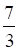B.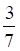C. –D. –Answer:Here, p(x) = 7x — 3To find the zeros of p(x), consider p(x) = 0∴ 7x — 3 = 0∴ x =Hence, the zero of the given polynomial is.The correct option is B.Question 11.The cubic polynomial p(x) = x3 — x has _____ zeros.A. 0B. 1C. 2D. 3Answer:Here, p(x) = x3 — xTo find the zeros of p(x), consider p(x) = 0∴x3 — x = 0∴ x (x2 – 1) = 0∴ x(x – 1)(x + 1) = 0 Using the identity: (a2 – b2) = (a – b) (a + b)∴ x = 0, x = 1, x = –1∴ The cubic polynomial has 3 zeros.The correct option is D.Question 12.The graph of p(x) = 3x — 2 — x2 intersects the X-axis in _____ points.A. 0B. 1C. 2D. 3Answer:The zeros of p(x) are the intersections points of the equation, p(x) = 3x – 2 – x2 with the x-axis.∴ The number of distinct zeros of p(x) gives the number of distinct intersection points on the X-axis.To find the zeros of p(x), consider p(x) = 0∴3x – 2 – x2 = 0∴ x2 – 3x+ 2 = 0∴ x2 – x – 2x+ 2 = 0∴ x(x – 1) – 2(x – 1) = 0∴ (x – 1)(x – 2) = 0∴ x = 1 or x = 2 are the zeros of p(x).⇒The graph of p(x) intersects X-axis at two points.The correct option is C.Question 13.The sum of the zeros of 3x2 + 5x — 2 is _____A.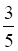B. –C.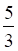D. –Answer:Given, p(x) = 3x2 + 5x — 2Here a = 3, b = 5, c = –2Sum of zeros, α + β = –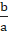= –The correct option is D.Question 14.The graph of p(x) = 3x + 5 represents _____A. a straight lineB. parabola open upwardsC. parabola open downwardsD. a rayAnswer:Given, p(x) = 3x+ 5Since p(x) is a linear polynomial, so its graph is a straight line.The correct option is A.Question 15.A quadratic polynomial has no zero. Its graph _____A. touches X-axis at any pointB. intersects X-axis at two distinct pointsC. does not intersect X-axis at two distinct pointsD. is in any one half plane of X-axisAnswer:The number of intersection points of a quadratic polynomial at X-axis is the number of its real zeros.Given, the quadratic polynomial has no zero.⇒ Its graph does not intersect X-axis or its graph lies in any one half plane of X-axis.The correct option is D.Question 16.For the graph in figure 2.11 y = p(x) has _____ zeros.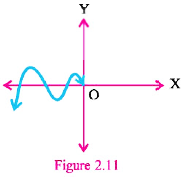A. 1B. 2C. 3D. 4Answer:The graph intersects the X-axis at four distinct points.⇒ p(x) has four zeros.The correct option is D.Question 17.The product of the zeros of x2 — 4x + 3 is _____A. 1B. 3C. 4D. —4Answer:Given, p(x) = x2 — 4x + 3Here a = 1, b = –4, c = 3Product of zeros, αβ == –= 3The correct option is B.Question 18.a = 3, b = 5, c = 7, d = 11 in the standard notation gives the cubic polynomial _____A. 3x3 + 5x2 — 7x — 11B. 3x3 — 5x2 + 7x — 11C. 3x3 + 5x2 — 7x + 11D. 3x3 + 5x2 + 7x + 11Answer:The standard form of a cubic polynomial is p(x) = ax3 + bx2 + cx + d∴ For a = 3, b = 5, c = 7 and d = 11,p(x) = 3x3 + 5x2 + 7x + 11.The correct option is D.

PDF FILE TO YOUR EMAIL IMMEDIATELY PURCHASE NOTES & PAPER SOLUTION. @ Rs. 50/- each (GST extra)

HINDI ENTIRE PAPER SOLUTION

MARATHI PAPER SOLUTION

SSC MATHS I PAPER SOLUTION

SSC MATHS II PAPER SOLUTION

SSC SCIENCE I PAPER SOLUTION

SSC SCIENCE II PAPER SOLUTION

SSC ENGLISH PAPER SOLUTION

SSC & HSC ENGLISH WRITING SKILL

HSC ACCOUNTS NOTES

HSC OCM NOTES

HSC ECONOMICS NOTES

HSC SECRETARIAL PRACTICE NOTES

# 2019 Board Paper Solution

HSC ENGLISH SET A 2019 21st February, 2019

HSC ENGLISH SET B 2019 21st February, 2019

HSC ENGLISH SET C 2019 21st February, 2019

HSC ENGLISH SET D 2019 21st February, 2019

SECRETARIAL PRACTICE (S.P) 2019 25th February, 2019

HSC XII PHYSICS 2019 25th February, 2019

CHEMISTRY XII HSC SOLUTION 27th, February, 2019

OCM PAPER SOLUTION 2019 27th, February, 2019

HSC MATHS PAPER SOLUTION COMMERCE, 2nd March, 2019

HSC MATHS PAPER SOLUTION SCIENCE 2nd, March, 2019

SSC ENGLISH STD 10 5TH MARCH, 2019.

HSC XII ACCOUNTS 2019 6th March, 2019

HSC XII BIOLOGY 2019 6TH March, 2019

HSC XII ECONOMICS 9Th March 2019

SSC Maths I March 2019 Solution 10th Standard11th, March, 2019

SSC MATHS II MARCH 2019 SOLUTION 10TH STD.13th March, 2019

SSC SCIENCE I MARCH 2019 SOLUTION 10TH STD. 15th March, 2019.

SSC SCIENCE II MARCH 2019 SOLUTION 10TH STD. 18th March, 2019.

SSC SOCIAL SCIENCE I MARCH 2019 SOLUTION20th March, 2019

SSC SOCIAL SCIENCE II MARCH 2019 SOLUTION, 22nd March, 2019

XII CBSE - BOARD - MARCH - 2019 ENGLISH - QP + SOLUTIONS, 2nd March, 2019

# HSCMaharashtraBoardPapers2020

(Std 12th English Medium)

HSC ECONOMICS MARCH 2020

HSC OCM MARCH 2020

HSC ACCOUNTS MARCH 2020

HSC S.P. MARCH 2020

HSC ENGLISH MARCH 2020

HSC HINDI MARCH 2020

HSC MARATHI MARCH 2020

HSC MATHS MARCH 2020

# SSCMaharashtraBoardPapers2020

(Std 10th English Medium)

English MARCH 2020

HindI MARCH 2020

Hindi (Composite) MARCH 2020

Marathi MARCH 2020

Mathematics (Paper 1) MARCH 2020

Mathematics (Paper 2) MARCH 2020

Sanskrit MARCH 2020

Sanskrit (Composite) MARCH 2020

Science (Paper 1) MARCH 2020

Science (Paper 2)

Geography Model Set 1 2020-2021

MUST REMEMBER THINGS on the day of Exam

Are you prepared? for English Grammar in Board Exam.

Paper Presentation In Board Exam

How to Score Good Marks in SSC Board Exams

Tips To Score More Than 90% Marks In 12th Board Exam

How to write English exams?

How to prepare for board exam when less time is left

How to memorise what you learn for board exam

No. 1 Simple Hack, you can try out, in preparing for Board Exam

How to Study for CBSE Class 10 Board Exams Subject Wise Tips?

JEE Main 2020 Registration Process – Exam Pattern & Important Dates

NEET UG 2020 Registration Process Exam Pattern & Important Dates

How can One Prepare for two Competitive Exams at the same time?

8 Proven Tips to Handle Anxiety before Exams!